# Hack 64. Spot Faked Data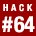If you haven't given it much thought before, it might be quite natural to assume that all digits are equally likely to show up in most random data sets. But according to Benford's law, for many types of naturally occurring data, the lower the digit, the more frequently it will occur as a leading digit. You can use this secret knowledge to check the authenticity of any data set.

In the 19th century, long before the age of electronic calculators, scientists used tables published in books to find values of logarithms. A particularly observant 19th-century astronomer and mathematician, Simon Newcomb, noticed that the pages of logarithm tables were more worn in the first pages than in the last pages. Newcomb concluded that numbers beginning with 1 occur more frequently than numbers beginning with 2, numbers beginning with 2 occur more frequently than numbers beginning with 3, and so on.

Newcomb published an empirical result based on his observations in the American Journal of Mathematics in 1881, which stated the probabilities of a number in many types of naturally occurring data, beginning with digit d for d = 1, 2, ... 9. Newcomb's first significant digit law received little attention and was largely forgotten until over 50 years later when Frank Benford, a physicist at General Electric, noticed the same pattern of wear and tear of logarithm tables.

After extensive testing (20,229 observations!) on a wide variety of dataincluding atomic weights, drainage areas of rivers, census figures, baseball statistics, and financial data, among other thingsBenford published the same probability law concerning the first significant digit in the Proceedings of the American Philosophical Society (Benford, 1938). This time, the first significant digit law attracted greater attention and became known as Benford's law. Although Benford's law became fairly well known after the 1938 paper, which included substantial statistical evidence, it lacked a rigorous mathematical foundation until that evidence was provided by Georgia Tech Mathematics professor Theodore Hill in 1996 (Hill, 1996).

Today, Benford's law is routinely applied in several areas in which naturally occurring data arise. Perhaps the most practical application of Benford's law is in detecting fraudulent data (or unintentional errors) in accounting, an application pioneered by Saint Michael's College Business Administration and Accounting professor Mark Nigrini (http://www.nigrini.com/).

The detection of fabricated data is important not only in accounting, but also in a wide variety of other applications (for example, clinical trials in drug testing). This hack describes Benford's law, shows you how to apply it, provides some intuitive justification on why it works, and gives some guidelines on when Benford's law can be applied.

#### How It Works

In its simplest form, Benford's law states that in many naturally occurring numerical data, the distribution of the first (nonzero) significant digit follows a logarithmic probability distribution described as follows. Following Hill (1997), let D 1(x) denote the first base 10 significant digit of a number x. For example, D 1(9108) = 9,and D 1(0.025108) = 2.

Then, according to Benford's law, the probability that D 1(x) = d, where d can equal 1, 2, 3, ..., 9, is given by the following equation:

Thus, Table 6-5 gives the probabilities of the first significant digits.

##### Table Probabilities of first digits under Benford's Law
First nonzero digitProbability according to Benford's law
10.301
20.176
30.125
40.097
50.079
60.067
70.058
80.051
90.046

#### Laying Down the Law

To demonstrate Benford's law, I'll consider two examples that you can verify yourself.

##### Street addresses

To see Benford's law in action, open the phone book of your city or town to any page, and record the number of house numbers that begin with each nonzero decimal digit. Two pages should be sufficient. Unless there is something very unusual about your town, the relative frequencies should resemble the respective probabilities predicted by Benford's law.

Table 6-6 shows results computed from the 413 house numbers taken from two pages of the 2005-2006 Narragansett/Newport/Westerly, RI Yellow Book (White Pages section).

##### Table Addresses following Benford's law
First nonzero digitRelative frequency for first digit of house number Probability according to Benford's law
10.3340.301
20.1740.176
30.1430.125
40.0750.097
50.0730.079
60.0750.067
70.0460.058
80.0430.051
90.0360.046

Figure 6-1 shows the pattern more clearly.

##### Figure 6-1. Street addresses following Benford's law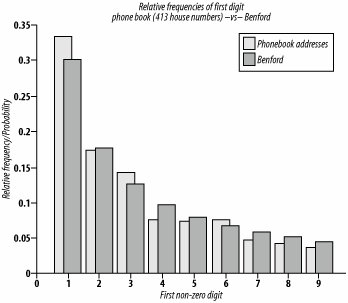Although the agreement with Benford's law is not perfect, you can see a reasonably good fit. If you take a larger sample of addresses, the resulting relative frequencies will be even closer to the probabilities predicted by Benford's law.

##### Stock prices

The stock market is known to follow Benford's law. You can verify this yourself by obtaining up-to-the-minute NASDAQ Securities prices at http://quotes.nasdaq.com/reference/comlookup.stm.

Figure 6-2 and Table 6-7 show the relative frequencies of the first nonzero decimal digits for NASDAQ Securities as of January 27, 2006, compared to the probabilities predicted by Benford's law.

##### Figure 6-2. The stock market following Benford's law##### Table NASDAQ securities following Benford's law
First nonzero digitRelative frequency for first digit of NASDAQ securitiesProbability according to Benford's law
10.3010.301
20.1670.176
30.1330.125
40.0950.097
50.0820.079
60.0710.067
70.0550.058
80.0450.051
90.0490.046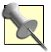You can obtain the Matlab code used to produce the tables and figures in this section at http://homepage.mac.com/samchops/benford/. Additionally, Mark Nigrini provides his DATAS software (including a free student EXCEL program), which performs a more sophisticated data analysis of the first, second, and first two digits, at http://www.nigrini.com/datas_software.htm.

#### More General Statements of Benford's Law

Benford's law does not apply to the first nonzero digit only, but also includes probabilities of other digits. Once again, following the treatment discussed earlier, let D 2(x)denote the second base-10 significant digit of a number x. For example, D 2(9108) = 1, D 2(9018) = 0, and D 1(0.025108) = 5. Notice that, unlike the first significant digit, the second significant digit can be zero.

Then, according to Benford's law, the probability that D 2(x) = d, where d can equal 0,1, 2, ..., 9, is given by the following equation:

This formula leads to the probabilities of the second significant digit, shown in Table 6-8.

##### Table Benford's second-digit law
Second significant digitProbability according to Benford's law
00.11968
10.11389
20.10882
30.10433
40.10031
50.09668
60.09337
70.09035
80.08757
90.08500

From Table 6-8, you can see that the differences among the probabilities of the second digit are not nearly as dramatic as those probabilities corresponding to the first digit.

Now, back to the stock market. To illustrate Benford's law as it relates to the second significant digit, I computed the relative frequencies of the second significant digits of our earlier NASDAQ Securities example. The results in Table 6-9 show, again, a close agreement with Benford's law.

##### Table NASDAQ securities following Benford's second-digit law
Second digitRelative frequency of second digitProbability according to Benford's law
00.128030.11968
10.114270.11389
20.109180.10882
30.102900.10433
40.102300.10031
50.092730.09668
60.090640.09337
70.091530.09035
80.084060.09035
90.084360.08500

A more general Benford's probability formula can be used to compute the respective probabilities of the nth digit. Let D k(x)denote the kth base-10 significant digit of a number x. Then, according to Benford's law, the probability that D 1(x)=d 1, D 2(x)=d 2,..., and D n(x)=d n is given by the following equation:

Note that if k does not equal 1, then d k can equal 0, 1, 2, ..., 9 and, as noted earlier, d 1 can equal 1, 2, ..., 9.

#### Where Else It Works

Two unique properties of Benford's Law are scale invariance and base invariance.

##### Scale invariance

Benford's law is scale-invariant; that is, if you multiply the data by any nonzero constant, you still wind up with a distribution that closely follows Benford's law. Thus, it makes no difference whether you measure stock quotes in dollars, dinars, or shekels, or whether you measure lengths of rivers in miles or kilometers. You'll always wind up with data that follows Benford's law.

To prove this, I took the NASDAQ securities data used in the earlier example and multiplied each value by p. As you can see in Table 6-10, the relative frequencies still follow Benford's law.

##### Table NASDAQ securities scaled by following Benford's law
First nonzero digitRelative frequency for first digit of NASDAQ securitiesProbability according to Benford's law
10.3060.301
20.1760.176
30.1230.125
40.0970.097
50.0810.079
60.0660.067
70.0580.058
80.0490.051
90.0450.046

##### Base invariance

The base-invariant property of Benford's law states that it applies not only in base 10, but also in more general bases. Moreover, Theodore Hill showed that Benford's law is the only probability law that has this property (Hill, 1995).You can find the formula for Benford's law in the general base-b case in Hill (1997). See the "See Also" section for publication details.

Benford's law works best on data that has the following characteristics:

Sufficient variability

The higher the variability, the better Benford's law applies.

No built-in maximum or other similar constraint

For example, Benford's law does not apply to the ages of high school seniors, or to members of the local senior citizen center.

Numbers that result from counting or measuring

For example, it does not work well for social security numbers and ZIP Codes, because they are simply identifiers and are not true numerical values.

Large sample size

The larger the data set, the better Benford's law applies.

Random sampling

The data results from a large number of random samples from a large number of randomly selected probability distributions. This realization by Hill led him to his proof of Benford's law (Becker, 2000; Hill, 1999).

Since tax data strongly follows Benford's law, it has been used quite successfully to identify fraudulent tax returns. In describing some of the basic features of Benford's law, we showed how anyone can perform a quick-and-dirty test for irregularities in data. Specifically, anyone can easily compute relative frequencies of first digits and eyeball the results juxtaposed with probabilities predicted by Benford's law.

In practice, the programs used by experts and authorities to identify deviations from Benford's law and other irregularities can be quite sophisticated. It is also important to keep in mind that deviation from Benford's law does not prove fraud, but it does raise red flags suggesting that further investigation might be indicated.For more details on the application of Benford's law to detect fraud, including a "goodness-of-fit" test, see Nigrini (1996). Consult this hack's "See Also" section for publication details.

#### Why It Works

Although the proof of Benford's law is quite technical, there are some insightful and intuitive explanations for this mathematical principle. One such explanation that I find particularly attractive has been provided by Mark Nigrini (1999).

His explanation goes something like this. If you imagine that some investment with an initial amount of \$100 is expected to grow at an annual rate of 10 percent, it would take about 7.3 years for the first digit of the total amount to change to 2. This is because the total amount has to increase by 100 percent to reach a value of \$200. In contrast, consider the time it would take for \$500 to increase to \$600. If we continue to assume an annual growth rate of 10 percent, it would take about 1.9 years to reach \$600. So, the amount of time until the investment amount has a first digit of 5 is considerably less that the amount of time it has a first digit of 1. Once the total amount reaches \$1,000, it will again take about 7.3 years before it will have a first digit of 2 (after another 100 percent increase).

The real world is a bit more complicated, but this does help to explain why 1 is a more common first digit than larger digits. Another intuitive explanation is that there are more small towns than large cities, and there are more short rivers than long rivers.

#### Where It Doesn't Work

Benford's law is less likely to apply in data sets with insufficient variability or data sets that are nonrandomly selected. For example, computer files sizes approximately follow Benford's law, but only if no restriction is placed on the type of files selected.

To illustrate this, I found the frequencies of the first digit of the file sizes on an Apple PowerBook G4. The results shown in Figure 6-3 and Table 6-11 exhibit the Benford's law pattern.

##### Figure 6-3. Computer files that follow Benford's law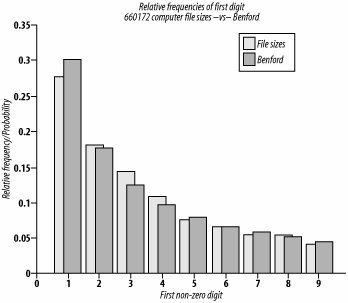##### Table Computer files that approximately follow Benford's law
First nonzero digitRelative frequency for first digit of 660,172 computer filesProbability according to Benford's law
10.2770.301
20.1810.176
30.1440.125
40.1070.097
50.0760.079
60.0670.067
70.0540.058
80.0540.051
90.0410.046

Although the results shown in Figure 6-3 and Table 6-11 are based on 660,172 files, Table 6-12 demonstrates that a sample size of 600 is large enough to exhibit the Benford's law pattern (albeit not as well as the larger sample), provided the sample of files is random.

##### Table Random selection of 600 computer files sizes
First nonzero digitRelative frequency for first digit of 600 computer filesProbability according to Benford's law
10.2620.301
20.1870.176
30.1470.125
40.1070.097
50.0690.079
60.0700.067
70.0520.058
80.0570.051
90.0520.046

For comparison, I computed the relative frequencies of MP3 files in an iTunes music library on the same computer. Table 6-13 and Figure 6-4 show that this set of files does not follow Benford's law.

##### Table Music MP3 files that do not follow Benford's law
First nonzero digitRelative frequency for first digit of 601 MP3 filesProbability according to Benford's law
10.0800.301
20.0970.176
30.2760.125
40.2700.097
50.1610.079
60.0700.067
70.0230.058
80.0130.051
90.0010.046

##### Figure 6-4. Music MP3 files that do not follow Benford's law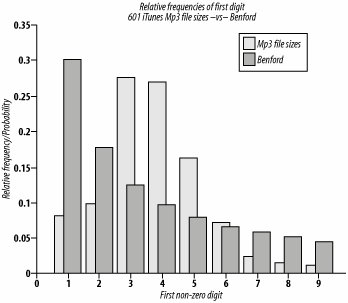The fact that the file sizes of about 600 MP3 music files do not approximate Benford's law is not surprising, since the sizes of MP3 music files exhibit much less variability than a more random selection of any 600 computer files.

#### See Also

• Becker, T. J. (2000). "Sorry, wrong number: Century-old math rule ferrets out modern-day digital deception," Georgia Tech Research Horizons, http://gtresearchnews.gatech.edu/reshor/rh-f00/math.html.

• Browne, M. (1998). "Following Benford's law, or looking out for no. 1." The New York Times, August 4, 1998.

• Fawcett, W. (n.d.). "Significant figure generator." http://williamfawcett.com/flash/SigFigDistbGen.htm.

• Benford, F. (1938). "The law of anomalous numbers." Proceedings of the American Philosophical Society, 78, 551-572.

• Hill, T. P. (1996). "A statistical derivation of the significant digit law." Statistical Science, 10, 354-363.

• Hill, T. P. (1995). "Base-invariance implies Benford's law." Proceedings of the American Mathematical Society, 123, 887-895.

• Hill, T. P. (1997). "Benford's law." Encyclopedia of Mathematics Supplement, 1, 112. Kluwer.

• Hill, T. P. (1999). "The difficulty of faking data." Chance, 26, 8-13.

• Newcomb, S. (1881). "Note on the frequency of use of the different digits in natural numbers." American Journal of Mathematics, 4, 72-40.

• Nigrini, M. (1999). "I've got your number: How a mathematical phenomenon can help CPAs uncover fraud and other irregularities." AICPA Journal of Accountancy Online Journal, May 1999, http://www.aicpa.org/pubs/jofa/may1999/nigrini.htm.

• Nigrini, M. (1996). "A taxpayer compliance application of Benford's law." Journal of the American Taxation Association, 18, 72-91.

• You can obtain the Matlab code used to produce the tables and figures in this section at http://homepage.mac.com/samchops/benford/. You'll need to have Matlab (http://www.mathworks.com) installed to run the code.

Ernest E. Rothman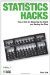Statistics Hacks: Tips & Tools for Measuring the World and Beating the Odds
ISBN: 0596101643
EAN: 2147483647
Year: 2004
Pages: 114
Authors: Bruce Frey

Similar book on Amazon

flylib.com © 2008-2017.
If you may any questions please contact us: flylib@qtcs.net